29Apr 2021

# Anatomy of a Deep learning Feedforward Neural Network used for Data Classification

In this article, we will explain how a typical Feedforward neural network works for data classification. A feedforward neural network is the simplest possible form of Deep learning Neural network.

We will go through all the steps involved in the classification and show how the data are transformed.

Here, data will be considered as 2D color maps with n x n pixels. Note that this image does not have to represent any geographical data and it can be generated from function plots.

## Using a ‘simple’ non-convolutional Network

In order to classify data, we can use at first a basic Artificial neural network with one hidden layer. E.g a multi-layer perception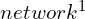or – in general – feed-forward neural network. For an n x n image, we will add a single hidden layer with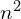neurons.

Here is our input image transformed as a source ofinput data.

We input the h pixels as a linear input of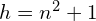neurons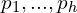. We use a hidden layer of j neurons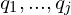. Finally, we put an output layer O1 which will classify the image in a binary way (e.g friend/foe for example)

As we know it we can use different activation functions like the sigmoid(Φ), tanh or linear activation functions. Here we will assume they are all the same, for the sake of simplicity.

## Training of the Network

We note P a pixel vector representing a data image. We note {P1*,d1},…,{Pk*,dk} the training data. This means that for the image represented by the pixel vector Pi* the output must be di.

We can back-propagate the result of each training using a simple delta rule, which is gradient descent using a least-square error, eg minimization of the quadratic difference between expected and obtained results.

For the k training data, the network will learn. Let us call wa,u1, a=1…h, u=1…j the weights (+bias) of the hidden layer and wb2, b=1…j the weights (+bias) of the output layer.

In our system, each layer will learn from the previous. This means that the j weights of the output layer will be re-adjusted first from the difference between the computed output and the desired output.

And then the weights from the hidden layer will be re-adjusted from the new values of the output layer (delta rule). And so on until all the data from the training set are processed.

Concretely wb2(new)=wb2(old)+r(d(i)-y(i))Xb(i), i=1…k, b=1…j

r is a positive constant <1 named the learning rate. y(i) is the output value O1 of the i-th sample and d(i) is the desired output.

Here, Xb(i) is the output of the b^th neuron of the hidden layer for the i^th sample of the training set.

The weights of the hidden layer are then adjusted by the same delta rule.

wa,b1(new)=wa,b1(old)+r(db(i)-zb(i))pa(i), i=1…k, a=1…h, b=1…j

zb(i) is the output value of the i-th sample of the b-th neuron qb of the hidden layer and db(i) is its desired output (eg the one computed in the i-th sample of the training set).

Recall that we can express Xb(i) by the formula:The output O1 is itself expressed byThis means that we have an overall formula for our neural network:Where pa(i) is the a^th pixel of the i^th sample and Φ the eventual activation function. Note that usually all data are normalized in the training data so that they fit in the range of [0.1].

Here we would consider normalized pixel values ofIf we do not wish to connect all the neurons of the input to all the neurons of the hidden layer, we simply set some weights to be zero.

We could have no hidden layer and opposingly we could add more hidden layers. The question is how does that change the accuracy of the neural networks and why.

Adding more hidden layers improves the efficiency of the neural network and reduces the error rate but the improvement itself decreases as the hidden layers are added while the computation time needed for training and operational use increases.

The efficiency of a neural network can be seen as a parallel computation with interconnected components which are “helping” each other. Then, intuitively, the more we add neurons that ‘helps’ the computation, the more accurate we must be.

Note that the delta rule we used does not always converge. It is preferable to use different rules sometimes such as “general” gradient descent methods.

## Geometrical Interpretation of the Classification

What the classifier does is to separate the input space, eg the n2 pixels represented as a discrete vector space of dimension n2, eg this can be seen as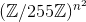.

Here It separates that space into two areas. The areas may not be connected. And if the data lies in one area it will be classified as dangerous/not dangerous etc.

There are several theorems of basic functional analysis that give a guarantee that such classification could be obtained under given specific conditions. We wish not to enter into the details.

Here we represent the output of a nonlinear feed-forward network. Each map is represented by a n2 coordinate. If the points lie in the region created by the classifier then it belongs to one category otherwise it belongs to the other category.

For this reason, convolutional networks are preferred. In the next article, we will perform the autopsy of the Lenet-1 convolutional network and how it can be used for data classification.

Acodez is a leading digital marketing agency in India. Our services includes SEO, SMM, SMO, PPC, and content marketing services to ensure that your website’s rank among the top results on the search engine. We are also a leading player in the website design company India arena, offering all kinds of web design and web development services at affordable prices. For further information, please contact us today.

## Looking for a good team for your next project?

on the web & mobile strategy that'd suit your needs best.